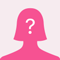# Help with math?Consider the Pythagorean theorem, distance formula , and equation of a circle with center at he origin and radius r. Explain how each of these formulas are related to each other.